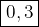## What is the y-intercept of this quadratic function f(x) = x2 + 2x + 3

Question

What is the y-intercept of this quadratic function f(x) = x2 + 2x + 3

in progress 0
3 months 2021-07-19T16:35:38+00:00 2 Answers 3 views 0

0,3

hope this helped !!!

Step-by-step explanation:

2. Hi there!Find the y-intercept by substituting in 0 for x:

f(0) = 0² + 2(0) + 3

Simplify:

f(0) = 3